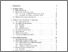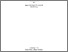## Group integrals in chaotic quantum systems

Kohler, Heiner

German Title: Gruppenintegrale in Chaotischen QuantensystemenPreview
PDF, English
Download (1MB) | Terms of usePreview
PDF, English
Download (88kB) | Terms of use

Citation of documents: Please do not cite the URL that is displayed in your browser location input, instead use the DOI, URN or the persistent URL below, as we can guarantee their long-time accessibility.

## Abstract

We derive a recursion formula for a class of group integrals both in ordinary space and in superspace. These group integrals represent the generalization of Bessel functions to matrix and supermatrix spaces. Thereby we derive exact expressions for the one and two--point eigenvalue correlator of a random matrix model. The model consists of a sum of two random matrices. One of them is diagonal and models the regular part. The other one is a Gaussian random matrix (GOE or GSE) modelling the chaotic admixture. We prove that in ordinary space the recursion formula is an integral solution of a Hamiltonian system related to Calogero--Sutherland models for arbitrary coupling beta>0. We calculate closed expressions for some group integrals over the unitary symplectic group. Moreover we generalize the Gelfand--Tzetlin coordinate system and construct a parametrization of the unitary orthosymplectic group UOSp(k_1/2k_2).

## Translation of abstract (English)

We derive a recursion formula for a class of group integrals both in ordinary space and in superspace. These group integrals represent the generalization of Bessel functions to matrix and supermatrix spaces. Thereby we derive exact expressions for the one and two--point eigenvalue correlator of a random matrix model. The model consists of a sum of two random matrices. One of them is diagonal and models the regular part. The other one is a Gaussian random matrix (GOE or GSE) modelling the chaotic admixture. We prove that in ordinary space the recursion formula is an integral solution of a Hamiltonian system related to Calogero--Sutherland models for arbitrary coupling beta>0. We calculate closed expressions for some group integrals over the unitary symplectic group. Moreover we generalize the Gelfand--Tzetlin coordinate system and construct a parametrization of the unitary orthosymplectic group UOSp(k_1/2k_2).

Item Type: Dissertation Guhr, Priv. Doz Thomas 6 December 2000 11 Jan 2001 00:00 2000 Service facilities > Max-Planck-Institute allgemein > MPI for Nuclear Physics 530 Physics Gruppentheorie, Quantenchaos, Supersymmetrie Dysons Brownsche Bewegung, Gruppenintegrale, Zufallsmatrixtheoriegroup integrals, quantum chaos, random matrix theory, supersymmetry